# Test Prep Plan - Take a practice test

Take this practice test to check your existing knowledge of the course material. We'll review your answers and create a Test Prep Plan for you based on your results.
How Test Prep Plans work
1
2Based on your results, we'll create a customized Test Prep Plan just for you!
3Study smarter
Study more effectively: skip concepts you already know and focus on what you still need to learn.

# Integral Calculus Chapter Exam

Exam Instructions:

Choose your answers to the questions and click 'Next' to see the next set of questions. You can skip questions if you would like and come back to them later with the yellow "Go To First Skipped Question" button. When you have completed the practice exam, a green submit button will appear. Click it to see your results. Good luck!

### Page 1

#### Question 1 1. Given f(x) on the graph below, which of the following integrals will equal 0?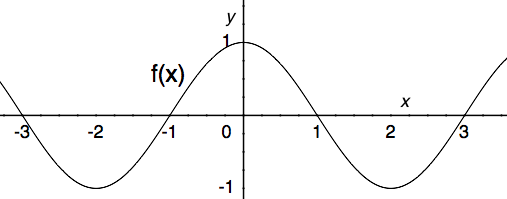#### Question 2 2. Which of the following graphs shows the correct region of integration for the integral below?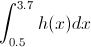#### Question 3 3. Given f(x) on the graph, what does z equal?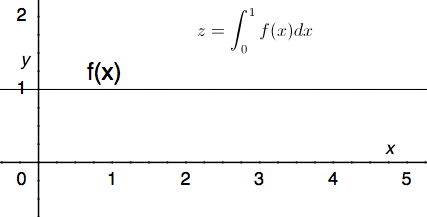#### Question 4 4. What is the two term left-sided Riemann Sum approximation for the integral below?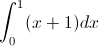#### Question 5 5. Which of the following is a representation of a Riemann sum estimation of the following integral?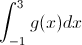### Page 2

#### Question 6 6. What is the one term left-sided Riemann Sum approximation for the integral below?#### Question 9 9. What is the curve length of f(x) between x=0 and x=1?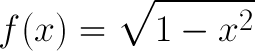#### Question 10 10. Evaluate the following integral.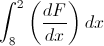### Page 3

#### Question 11 11. Which of the following shows the correct method for evaluating the integral below?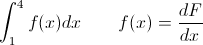#### Question 12 12. Which integral would be evaluated using the following expression (given that F'(x) = f(x))?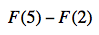#### Question 13 13. Evaluate the following.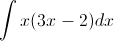#### Question 14 14. Evaluate the following integral.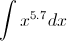#### Question 15 15. Calculate the integral.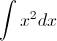### Page 4

#### Question 16 16. Integrate the following.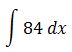#### Question 17 17. Integrate the following.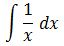#### Question 18 18. Integrate the following.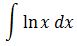#### Question 19 19. Evaluate the following.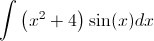#### Question 20 20. Use integration by parts to integrate the following: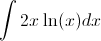### Page 5

#### Question 21 21. Which of the following is the BEST way to solve the integral below using integration by parts?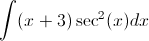#### Question 22 22. Evaluate the following integral.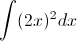#### Question 23 23. Evaluate the following integral.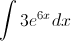#### Question 24 24. Evaluate the following integral.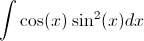#### Question 25 25. Which of the following is your start point?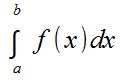### Page 6

#### Question 26 26. Evaluate.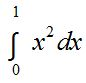#### Integral Calculus Chapter Exam Instructions

Choose your answers to the questions and click 'Next' to see the next set of questions. You can skip questions if you would like and come back to them later with the yellow "Go To First Skipped Question" button. When you have completed the practice exam, a green submit button will appear. Click it to see your results. Good luck!

Support